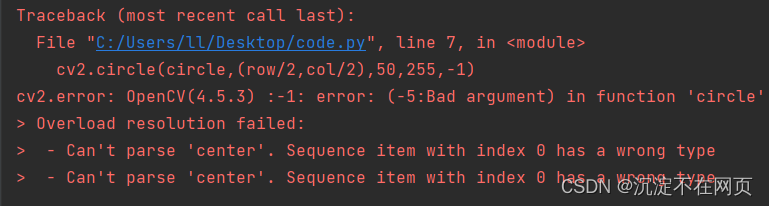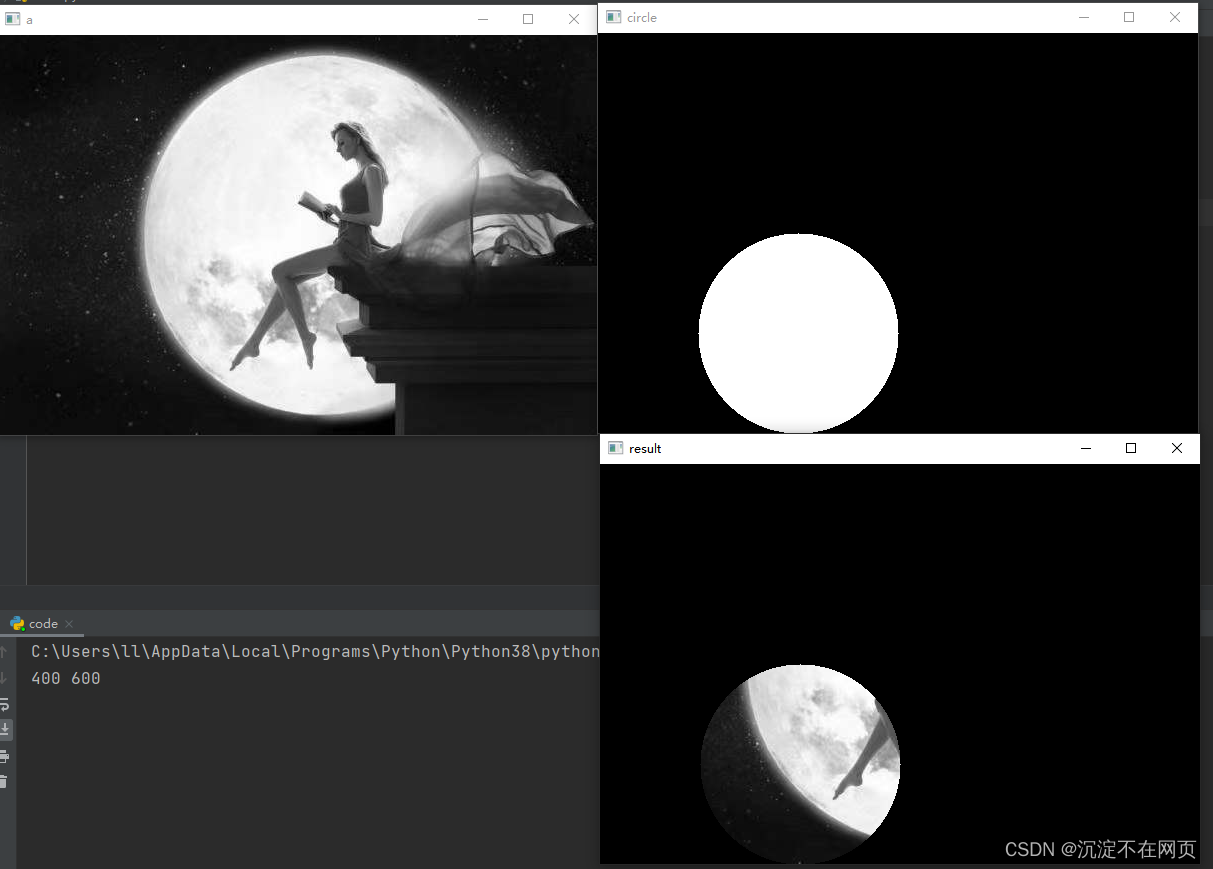# [Solved] cv2.error: OpenCV(4.5.3) :-1: error: (-5:Bad argument) in function ‘circle‘

Here, I operate on the image

``````import cv2
import numpy as np
row,col=img.shape[:2]
print(row,col)
circle=np.zeros((row,col),dtype='uint8')
cv2.circle(circle,(row/2,col/2),100,255,-1)
result=cv2.bitwise_and(img,circle)
cv2.imshow('a',img)
cv2.imshow('circle',circle)
cv2.imshow('result',result)
cv2.waitKey(0)
cv2.destroyAllWindows()
``````

An error is found during operation, as shown in the following figureThe error reported is a type error, which makes it impossible to analyze the coordinates of the center of the circle. This is mainly because our center coordinate is obtained by dividing the width and height of the picture by two. It is of float type, and the others are of int type. We can convert the center coordinate type to int type

``cv2.circle(circle,(row//2,col//2),50,255,-1)``

The results after operation are shown in the following figure: